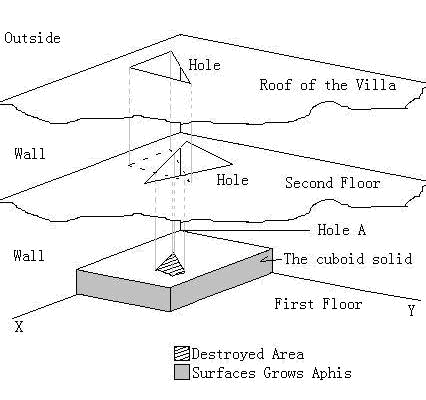POJ3591 :Ants and Aphides

There is a big villa far away from the city, and no one lived there for a long time. In the room on the first floor, ants have already built their community under a fixed cuboid solid in the corner of this room, which is illustrated in Figure 1.Figure 1
There is a hole at Point A which is the entrance and exit of the ants' nest and the ants will start their aphis explorer here. Aphides grow on the vertical surfaces of the cuboid solid. Everyday the ants go out to one of the aphides and take it back. The ants can go to the aphis directly if they know where it grows though they may not see it.
It's dangerous somewhere in the villa! For the perennial acid rain in this area, and also no one came to maintain this villa for years, all the floors of the villa have big holes.
As you see in Figure 1, the acid rain has destroyed somewhere on the roof of the solid. Just image, the destroyed area is the only area on the cuboid roof surface where you can see the sky when you looking up. Thus, when the ants are out to fetch aphides they will keep away from the acid-destroyed area.
For simplicity, we assume that:
The aphides do not move.
Each floor has only one hole.
All the holes on the floors are regular convex polygons.
The acid rain falls strictly vertically in the villa.
The brim of the acid-destroyed area is safe for the ants.
The aphis ants will not dig holes for fetching aphides.
Now give the coordinate of ONE aphis and help the ants to calculate the shortest path to get to it.

The first line of the input is an integer T which indicates the number of test cases.
Each of the case, the first line will be the three integers L, W, and H, (0 < L, W, H ≤ 10000 indicate the length (x-coordinate), width (y-coordinate) and the height (z-coordinate) of the solid.
The next line follows an integer K (0 ≤ K ≤ 10), indicating the number of floors with holes above the solid (including the roof of the villa). Then follows K lines describe the holes. Each of the K lines begin with an integer indicating the number Ni (3 ≤ Ni ≤ 100) of points of the convex polygons of the hole, the next Ni pairs of integers will be the continuous points of the hole counter-clockwise, you can assume that all the coordinates of the points are in the range (0, 10000). The floors are all horizontal and you need not to know exactly how high they are.
Then follows a three-dimensional integer coordinate (x, y, z) indicating the location of the target aphis. You can assume that this point is always on the plane X = L or Y = W.
The original point of the coordinate system is inside the solid, and the coordinate of Point A is (0, 0, H).

Output a real number with a precision of three decimals of the shortest path for each test case.

1
10 10 10
2
4 2 1 8 1 8 8 2 8
4 2 2 8 2 8 9 2 9
10 10 0

22.668

1个回答